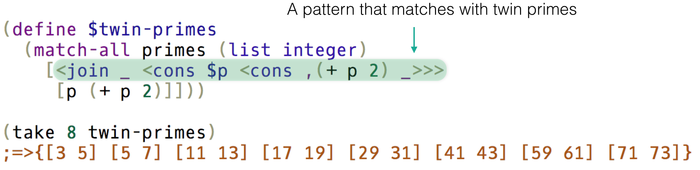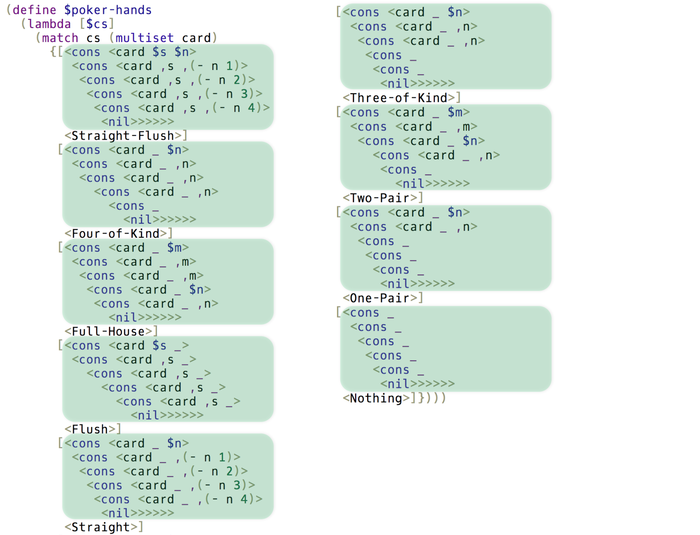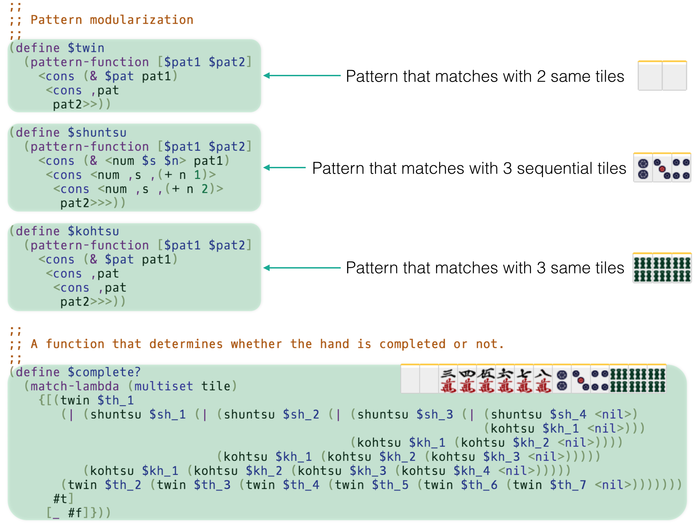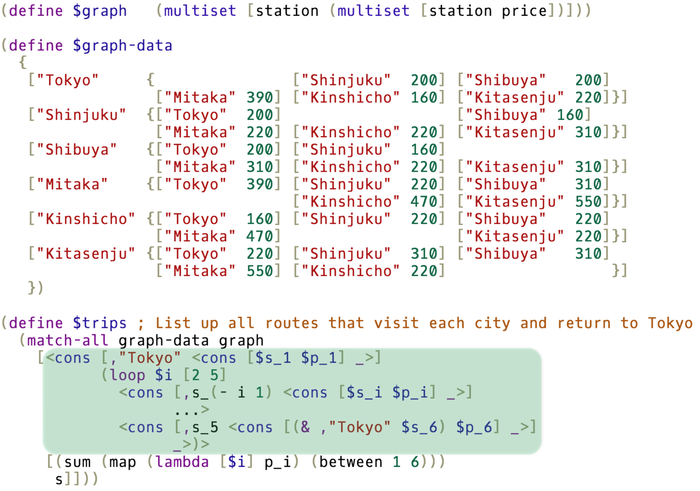# egison: Programming language with non-linear pattern-matching against non-free data

[ compilers-interpreters, library, mit, program ] [ Propose Tags ]

An interpreter for Egison, a **pattern-matching-oriented**, purely functional programming language. We can directly represent pattern-matching against lists, multisets, sets, trees, graphs and any kind of data types.

We can find Egison programs in lib and sample directories. This package also include Emacs Lisp file elisp/egison-mode.el.

We can do non-linear pattern-matching against non-free data types in Egison. An non-free data type is a data type whose data have no canonical form, a standard way to represent that object. It enables us to write elegant programs.

Twin Primes

We can use pattern-matching for enumeration. The following code enumerates all twin primes from the infinite list of prime numbers with pattern-matching!Poker Hands

The following code is the program that determines poker-hands written in Egison. All hands are expressed in a single pattern.Mahjong

We can write a pattern even against mahjong tiles. We modularize patterns to represent complex mahjong hands.Graphs

We can pattern-match against graphs. We can write program to solve the travelling salesman problem in a single pattern-matching expression.Aren't thsese exciting? We can run these demonstrations on our website! Please view and try more demonstrations!

http://www.egison.org

Egison makes programming dramatically simple. Please enjoy Egison!

Versions [faq] 0.1, 0.1.1, 0.1.2, 0.1.2.1, 0.1.2.2, 0.1.2.3, 0.1.2.4, 0.1.2.5, 0.2.0.0, 0.2.0.1, 0.2.0.2, 0.2.1.0, 0.2.1.1, 0.3.0.0, 0.3.0.1, 0.3.0.2, 0.3.0.3, 0.3.1.0, 0.3.1.1, 0.4.0.0, 1.0, 1.0.1, 1.0.2, 1.0.3, 1.0.4, 1.0.5, 1.0.6, 1.0.7, 1.1.0, 1.1.1, 1.2.0, 1.2.1, 1.2.2, 1.2.3, 2.0.0, 2.0.1, 2.0.2, 2.0.3, 2.0.4, 2.1.0, 2.1.1, 2.1.2, 2.1.3, 2.1.4, 2.1.5, 2.1.6, 2.1.7, 2.1.8, 2.1.9, 2.1.10, 2.1.11, 2.1.12, 2.1.13, 2.1.14, 2.1.15, 2.1.16, 2.2.0, 2.2.1, 2.2.2, 2.2.3, 2.3.0, 2.3.1, 2.3.2, 2.3.3, 2.3.4, 2.3.5, 2.3.6, 2.3.7, 2.3.8, 2.3.9, 2.3.10, 2.4.0, 2.4.1, 2.4.2, 2.4.3, 2.4.4, 2.4.5, 2.4.6, 2.4.7, 3.0.0, 3.0.1, 3.0.2, 3.0.3, 3.0.4, 3.0.5, 3.0.6, 3.0.7, 3.0.8, 3.0.9, 3.0.10, 3.0.11, 3.0.12, 3.1.0, 3.1.1, 3.2.0, 3.2.1, 3.2.2, 3.2.3, 3.2.4, 3.2.5, 3.2.6, 3.2.7, 3.2.8, 3.2.9, 3.2.10, 3.2.11, 3.2.12, 3.2.13, 3.2.14, 3.2.15, 3.2.16, 3.2.17, 3.2.18, 3.2.19, 3.2.20, 3.2.21, 3.2.22, 3.2.23, 3.2.24, 3.3.0, 3.3.1, 3.3.2, 3.3.3, 3.3.4, 3.3.5, 3.3.6, 3.3.7, 3.3.8, 3.3.9, 3.3.10, 3.3.11, 3.3.12, 3.3.13, 3.3.14, 3.3.15, 3.3.16, 3.3.17, 3.4.0, 3.5.0, 3.5.1, 3.5.2, 3.5.3, 3.5.4, 3.5.5, 3.5.6, 3.5.7, 3.5.8, 3.5.9, 3.5.10, 3.6.0, 3.6.1, 3.6.2, 3.6.3, 3.6.4, 3.6.5, 3.7.0, 3.7.1, 3.7.2, 3.7.3, 3.7.4, 3.7.5, 3.7.6, 3.7.7, 3.7.8, 3.7.9, 3.7.10, 3.7.11, 3.7.12, 3.7.13, 3.7.14, 3.8.0, 3.8.1, 3.8.2, 3.9.0, 3.9.1, 3.9.2, 3.9.3, 3.9.4, 3.10.0, 3.10.1, 3.10.2, 3.10.3, 4.0.0, 4.0.1, 4.0.2, 4.0.3, 4.1.0, 4.1.1, 4.1.2 Changelog.md array, base (>=4.7 && <5), containers, directory, egison, exceptions, fail, filepath, ghc, ghc-paths, hashable, haskeline, megaparsec (>=7.0.0), mtl (>=2.2.1), optparse-applicative, parsec (>=3.1), parser-combinators, prettyprinter, process, random, regex-tdfa, semigroups, split, sweet-egison (>=0.1.1.3), text, transformers, unicode-show, unordered-containers, vector [details] MIT Satoshi Egi, Ryo Tanaka, Takahisa Watanabe, Kentaro Honda, Mayuko Kori, Momoko Hattori Satoshi Egi Compilers/Interpreters http://www.egison.org head: git clone https://github.com/egison/egison.git by SatoshiEgi at 2020-10-26T03:25:00Z NixOS:4.1.1 egison-translate, egison 136725 total (100 in the last 30 days) 2.0 (votes: 1) [estimated by Bayesian average] λ λ λDocs not available All reported builds failed as of 2020-10-26

## Modules

• Language
• Language.Egison
• Language.Egison.AST
• Language.Egison.CmdOptions
• Language.Egison.Completion
• Language.Egison.Core
• Language.Egison.Data
• Language.Egison.Data.Collection
• Language.Egison.Data.Utils
• Language.Egison.Desugar
• Language.Egison.Eval
• Language.Egison.EvalState
• Language.Egison.IExpr
• Language.Egison.MList
• Language.Egison.Match
• Language.Egison.Math
• Language.Egison.Math.Arith
• Language.Egison.Math.Expr
• Language.Egison.Math.Normalize
• Language.Egison.Math.Rewrite
• Language.Egison.MathOutput
• Language.Egison.Parser
• Language.Egison.Parser.NonS
• Language.Egison.Parser.SExpr
• Language.Egison.Pretty
• PrettyMath
• Language.Egison.PrettyMath.AST
• Language.Egison.PrettyMath.AsciiMath
• Language.Egison.PrettyMath.Latex
• Language.Egison.PrettyMath.Mathematica
• Language.Egison.PrettyMath.Maxima
• Language.Egison.Primitives
• Language.Egison.Primitives.Arith
• Language.Egison.Primitives.IO
• Language.Egison.Primitives.String
• Language.Egison.Primitives.Types
• Language.Egison.Primitives.Utils
• Language.Egison.RState
• Language.Egison.Tensor

#### Maintainer's Corner

For package maintainers and hackage trustees

[back to package description]

# The Egison Programming LanguageEgison is a functional programming language featuring its expressive pattern-matching facility. Egison allows users to define efficient and expressive pattern-matching methods for arbitrary user-defined data types including non-free data types such as lists, multisets, sets, trees, graphs, and mathematical expressions. This is the repository of the interpreter of Egison.

## Non-Linear Pattern Matching for Non-Free Data Types

We can use non-linear pattern matching for non-free data types in Egison. A non-free data type is a data type whose data have no canonical form, or a standard way to represent that object. For example, multisets are non-free data types because a multiset {a,b,b} has two other syntastically different representations: {b,a,b} and {b,b,a}. Expressive pattern matching for these data types enables us to write elegant programs.

### Twin Primes

We can use pattern matching for enumeration. The following code enumerates all twin primes from the infinite list of prime numbers with pattern matching!

def twinPrimes :=
matchAll primes as list integer with
| _ ++ $p :: #(p + 2) :: _ -> (p, p + 2) take 8 twinPrimes -- [(3, 5), (5, 7), (11, 13), (17, 19), (29, 31), (41, 43), (59, 61), (71, 73)]  ### Poker Hands The following code is a program that determines poker-hands written in Egison. All hands are expressed in a single pattern. def poker cs := match cs as multiset card with | card$s $n :: card #s #(n-1) :: card #s #(n-2) :: card #s #(n-3) :: card #s #(n-4) :: _ -> "Straight flush" | card _$n :: card _ #n :: card _ #n :: card _ #n :: _ :: []
-> "Four of a kind"
| card _ $m :: card _ #m :: card _ #m :: card _$n :: card _ #n :: []
-> "Full house"
| card $s _ :: card #s _ :: card #s _ :: card #s _ :: card #s _ :: [] -> "Flush" | card _$n :: card _ #(n-1) :: card _ #(n-2) :: card _ #(n-3) :: card _ #(n-4) :: []
-> "Straight"
| card _ $n :: card _ #n :: card _ #n :: _ :: _ :: [] -> "Three of a kind" | card _$m :: card _ #m :: card _ $n :: card _ #n :: _ :: [] -> "Two pair" | card _$n :: card _ #n :: _ :: _ :: _ :: []
-> "One pair"
| _ :: _ :: _ :: _ :: _ :: [] -> "Nothing"


### Graphs

We can pattern-match against graphs. We can write a program to solve the travelling salesman problem in a single pattern-matching expression.

def graph := multiset (string, multiset (string, integer))

def graphData :=
[("Berlin", [("New York", 14), ("London", 2), ("Tokyo", 14), ("Vancouver", 13)]),
("New York", [("Berlin", 14), ("London", 12), ("Tokyo", 18), ("Vancouver", 6)]),
("London", [("Berlin", 2), ("New York", 12), ("Tokyo", 15), ("Vancouver", 10)]),
("Tokyo", [("Berlin", 14), ("New York", 18), ("London", 15), ("Vancouver", 12)]),
("Vancouver", [("Berlin", 13), ("New York", 6), ("London", 10), ("Tokyo", 12)])]

def trips :=
let n := length graphData in
matchAll graphData as graph with
| (#"Berlin", (($s_1,$p_1) : _)) ::
loop $i (2, n - 1) ((#s_(i - 1), ($s_i, $p_i) :: _) :: ...) ((#s_(n - 1), (#"Berlin" &$s_n, $p_n) :: _) :: []) -> sum (map (\i -> p_i) [1..n]), map (\i -> s_i) [1..n] car (sortBy (\(_, x), (_, y) -> compare x y)) trips) -- (["London", "New York", "Vancouver", "Tokyo"," Berlin"], 46)  ## Egison as a Computer Algebra System As an application of Egison pattern matching, we have implemented a computer algebra system on Egison. The most part of this computer algebra system is written in Egison and extensible using Egison. ### Symbolic Algebra Egison treats unbound variables as symbols. > x x > (x + y)^2 x^2 + 2 * x * y + y^2 > (x + y)^4 x^4 + 4 * x^3 * y + 6 * x^2 * y^2 + 4 * x * y^3 + y^4  We can handle algebraic numbers, too. > sqrt x sqrt x > sqrt 2 sqrt 2 > x + sqrt y x + sqrt y  ### Complex Numbers The symbol i is defined to rewrite i^2 to -1 in Egison library. > i * i -1 > (1 + i) * (1 + i) 2 * i > (x + y * i) * (x + y * i) x^2 + 2 * x * y * i - y^2  ### Square Root The rewriting rule for sqrt is also defined in Egison library. > sqrt 2 * sqrt 2 2 > sqrt 6 * sqrt 10 2 * sqrt 15 > sqrt (x * y) * sqrt (2 * x) x * sqrt 2 * sqrt y  ### The 5th Roots of Unity The following is a sample to calculate the 5th roots of unity. > qF' 1 1 (-1) ((-1 + sqrt 5) / 2, (-1 - sqrt 5) / 2) > def t := fst (qF' 1 1 (-1)) > qF' 1 (-t) 1 ((-1 + sqrt 5 + sqrt 2 * sqrt (-5 - sqrt 5)) / 4, (-1 + sqrt 5 - sqrt 2 * sqrt (-5 - sqrt 5)) / 4) > def z := fst (qF' 1 (-t) 1) > z (-1 + sqrt 5 + sqrt 2 * sqrt (-5 - sqrt 5)) / 4 > z ^ 5 1  ### Differentiation We can implement differentiation easily in Egison. > d/d (x ^ 3) x 3 * x^2 > d/d (e ^ (i * x)) x exp (x * i) * i > d/d (d/d (log x) x) x -1 / x^2 > d/d (cos x * sin x) x -2 * (sin x)^2 + 1  ### Taylor Expansion The following sample executes Taylor expansion on Egison. We verify Euler's formula in the following sample. > take 8 (taylorExpansion (exp (i * x)) x 0) [1, x * i, - x^2 / 2, - x^3 * i / 6, x^4 / 24, x^5 * i / 120, - x^6 / 720, - x^7 * i / 5040] > take 8 (taylorExpansion (cos x) x 0) [1, 0, - x^2 / 2, 0, x^4 / 24, 0, - x^6 / 720, 0] > take 8 (taylorExpansion (i * sin x) x 0) [0, x * i, 0, - x^3 * i / 6, 0, x^5 * i / 120, 0, - x^7 * i / 5040] > take 8 (map2 (+) (taylorExpansion (cos x) x 0) (taylorExpansion (i * sin x) x 0)) [1, x * i, - x^2 / 2, - x^3 * i / 6, x^4 / 24, x^5 * i / 120, - x^6 / 720, - x^7 * i / 5040]  ### Tensor Index Notation Egison supports tesnsor index notation. We can use Einstein notation to express arithmetic operations between tensors. The method for importing tensor index notation into programming is discussed in Egison tensor paper. The following sample is from Riemann Curvature Tensor of S2 - Egison Mathematics Notebook. -- Parameters def x := [| θ, φ |] def X := [| r * (sin θ) * (cos φ) -- x , r * (sin θ) * (sin φ) -- y , r * (cos θ) -- z |] def e_i_j := (∂/∂ X_j x~i) -- Metric tensors def g_i_j := generateTensor (\x y -> V.* e_x_# e_y_#) [2, 2] def g~i~j := M.inverse g_#_# g_#_# -- [| [| r^2, 0 |], [| 0, r^2 * (sin θ)^2 |] |]_#_# g~#~# -- [| [| 1 / r^2, 0 |], [| 0, 1 / (r^2 * (sin θ)^2) |] |]~#~# -- Christoffel symbols def Γ_i_j_k := (1 / 2) * (∂/∂ g_i_k x~j + ∂/∂ g_i_j x~k - ∂/∂ g_j_k x~i) Γ_1_#_# -- [| [| 0, 0 |], [| 0, -1 * r^2 * (sin θ) * (cos θ) |] |]_#_# Γ_2_#_# -- [| [| 0, r^2 * (sin θ) * (cos θ) |], [| r^2 * (sin θ) * (cos θ), 0 |] |]_#_# def Γ~i_j_k := withSymbols [m] g~i~m . Γ_m_j_k Γ~1_#_# -- [| [| 0, 0 |], [| 0, -1 * (sin θ) * (cos θ) |] |]_#_# Γ~2_#_# -- [| [| 0, (cos θ) / (sin θ) |], [| (cos θ) / (sin θ), 0 |] |]_#_# -- Riemann curvature def R~i_j_k_l := withSymbols [m] ∂/∂ Γ~i_j_l x~k - ∂/∂ Γ~i_j_k x~l + Γ~m_j_l . Γ~i_m_k - Γ~m_j_k . Γ~i_m_l R~#_#_1_1 -- [| [| 0, 0 |], [| 0, 0 |] |]~#_# R~#_#_1_2 -- [| [| 0, (sin θ)^2 |], [| -1, 0 |] |]~#_# R~#_#_2_1 -- [| [| 0, -1 * (sin θ)^2 |], [| 1, 0 |] |]~#_# R~#_#_2_2 -- [| [| 0, 0 |], [| 0, 0 |] |]~#_#  ### Differential Forms By designing the index completion rules for omitted indices, we can use the above notation to express a calculation handling the differential forms. The following sample is from Curvature Form - Egison Mathematics Notebook. -- Parameters and metric tensor def x := [| θ, φ |] def g_i_j := [| [| r^2, 0 |], [| 0, r^2 * (sin θ)^2 |] |]_i_j def g~i~j := [| [| 1 / r^2, 0 |], [| 0, 1 / (r^2 * (sin θ)^2) |] |]~i~j -- Christoffel symbols def Γ_j_l_k := (1 / 2) * (∂/∂ g_j_l x~k + ∂/∂ g_j_k x~l - ∂/∂ g_k_l x~j) def Γ~i_k_l := withSymbols [j] g~i~j . Γ_j_l_k -- Exterior derivative def d %t := !(flip ∂/∂) x t -- Wedge product infixl expression 7 ∧ def (∧) %x %y := x !. y -- Connection form def ω~i_j := Γ~i_j_# -- Curvature form def Ω~i_j := withSymbols [k] antisymmetrize (d ω~i_j + ω~i_k ∧ ω~k_j) Ω~#_#_1_1 -- [| [| 0, 0 |], [| 0, 0 |] |]~#_# Ω~#_#_1_2 -- [| [| 0, (sin θ)^2 / 2|], [| -1 / 2, 0 |] |]~#_# Ω~#_#_2_1 -- [| [| 0, -1 * (sin θ)^2 / 2 |], [| 1 / 2, 0 |] |]~#_# Ω~#_#_2_2 -- [| [| 0, 0 |], [| 0, 0 |] |]~#_#  ### Egison Mathematics Notebook Here are more samples. ## Comparison with Related Work There are <a target="_blank" href="https://ghc.haskell.org/trac/ghc/wiki/ViewPatterns#Relatedwork">a lot of existing work</a> for pattern matching. The advantage of Egison is that it fulfills the following two requirements at the same time. 1. Efficient backtracking algorithm for non-linear pattern matching. 2. Extensibility of patterns. Additionally, it fulfills the following requirements. 1. Polymorphism of patterns. 2. Pattern matching with infinitely many results. Check out <a target="_blank" href="https://arxiv.org/abs/1808.10603">our paper</a> for details. ## Installation Installation guide is available on our website. If you are a beginner of Egison, it would be better to install <a target="_blank" href="https://github.com/egison/egison-tutorial">egison-tutorial</a> as well. We also have online interpreter and online tutorial. Enjoy! ## Notes for Developers You can build Egison as follows: $ stack init
\$ stack build --fast